# Partial function evaluation in pirouette

This vignette describes partial function evaluation used in pirouette.

library(pirouette)

We’ll use pryr (from the Tidyverse to get that functionality.

library(pryr)

## The functions

These are the functions we’ll create, in order of increasing complexity:

1. Create a twin tree
2. Create a true alignment
3. Create a twin alignment

## 1. create a twin tree

We start from a simple true tree:

true_phylogeny <- ape::read.tree(text = "((A:1, B:1):1, C:2);")
ape::plot.phylo(true_phylogeny, main = "True phylogeny")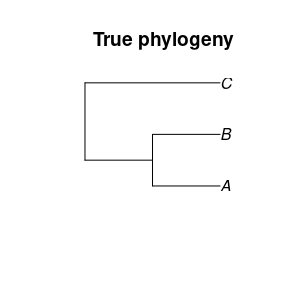To create a twin tree, I will describe:

• 1.1 Create a twin tree using the defaults
• 1.2 Create a twin tree using a built-in BD model
• 1.3 Create a twin tree using a custom function
• 1.4 Create a twin tree using a custom function with more arguments
• 1.5 Create a twin tree using a built-in function with more arguments that uses the Yule speciation model
• 1.6 Create a twin tree using a built-in function to copy a tree

### 1.1 Create a twin tree using the defaults

To create a twin tree from the true tree using the default settings is easily done by calling create_twin_tree:

twin_tree <- pirouette::create_twin_tree(true_phylogeny)
ape::plot.phylo(twin_tree, main = "Twin phylogeny")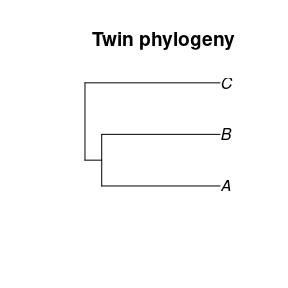The create_twin_tree function creates the twin tree in the way specified in its other function argument: the twinning_params:

twinning_params <- pirouette::create_twinning_params()

Calling create_twin_tree results in the same twin tree (yes, go ahead and check!):

twin_tree <- pirouette::create_twin_tree(
phylogeny = true_phylogeny,
twinning_params = twinning_params
)
ape::plot.phylo(twin_tree, main = "Twin phylogeny")## 1.2 Create a twin tree using a built-in BD model

How to specify how the twin tree is created? Set the value for the twinning_params$sim_twin_tree_fun! I’ll demonstrate using the get_sim_bd_twin_tree_fun which produces a function that simulates a twin tree using a Birth-Death speciation model: twinning_params <- pirouette::create_twinning_params( sim_twin_tree_fun = pirouette::get_sim_bd_twin_tree_fun() ) Proceed as usual here to simulate the twin tree: twin_tree <- pirouette::create_twin_tree( phylogeny = true_phylogeny, twinning_params = twinning_params ) ape::plot.phylo(twin_tree, main = "Twin phylogeny")### 1.3 Create a twin tree using a custom function twinning_params$sim_twin_tree_fun must be a function that takes one argument, which must be the true phylogeny.

Here is an example that creates a twin tree by simulation a random coalescent tree with the same number of tips:

# Define my function
my_fun <- function(true_phylogeny) {
new_phylo <- ape::rcoal(n = ape::Ntip(true_phylogeny))
new_phylo$tip.label <- true_phylogeny$tip.label # nolint ape style, not mine
new_phylo
}
# Put my function in the twinning_params
twinning_params <- pirouette::create_twinning_params(
sim_twin_tree_fun = my_fun
)
# Create a twin tree using my function
twin_tree <- pirouette::create_twin_tree(
phylogeny = true_phylogeny,
twinning_params = twinning_params
)
# Show the twin tree created by my function
ape::plot.phylo(twin_tree, main = "Twin phylogeny")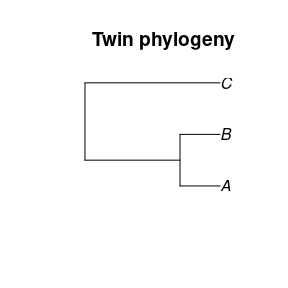### 1.4 Create a twin tree using a built-in function with more arguments

So, twinning_params$sim_twin_tree_fun must be a function that takes one argument, which is the true phylogeny. What if we want a function that indeed has one argument for the true phylogeny, but also some additional ones? An example is sim_bd_twin_tree, which uses a method and a number of replicates: head(sim_bd_twin_tree) ## ## 1 function (true_phylogeny, method = "random_tree", n_replicates = 10000, ## 2 os = rappdirs::app_dir()$os)
## 3 {
## 4     beastier::check_os(os)
## 5     methods <- c("random_tree", "max_clade_cred", "max_likelihood")
## 6     if (!method %in% methods) {

We cannot simply plug it in:

tryCatch(
pirouette::create_twinning_params(
sim_twin_tree_fun = sim_bd_twin_tree
),
error = function(e) {
cat(emessage) } ) ## Warning in if (stringr::str_count(string = arguments, pattern = ",") > 0) {: the ## condition has length > 1 and only the first element will be used ## 'sim_twin_tree_fun' must be a function with one argument Instead, we’ll need partial function evaluation, which will create a function with one a ellipsis (...) argument that will fill in the values you specified: # Create my partially evaluated function sim_twin_tree_fun <- pryr::partial( sim_bd_twin_tree, method = "random_tree", n_replicates = 1 ) # Create twinning_params with my function twinning_params <- pirouette::create_twinning_params( sim_twin_tree_fun = sim_twin_tree_fun ) # Create a twin tree using my function twin_tree <- pirouette::create_twin_tree( phylogeny = true_phylogeny, twinning_params = twinning_params ) # Show the twin tree ape::plot.phylo(twin_tree, main = "Twin phylogeny")There is a helper function called get_sim_bd_twin_tree_fun that does the same partial function evaluation for you: # Create twinning_params with my function twinning_params <- pirouette::create_twinning_params( sim_twin_tree_fun = pirouette::get_sim_bd_twin_tree_fun( method = "random_tree", n_replicates = 1 ) ) # Create a twin tree using my function twin_tree <- pirouette::create_twin_tree( phylogeny = true_phylogeny, twinning_params = twinning_params ) # Show the twin tree ape::plot.phylo(twin_tree, main = "Twin phylogeny")### 1.5 Create a twin tree using a built-in function with more arguments that uses the Yule speciation model Now using a Yule model: # Create twinning_params with my function twinning_params <- pirouette::create_twinning_params( sim_twin_tree_fun = pirouette::create_sim_yule_twin_tree_fun( method = "random_tree", n_replicates = 1 ) ) # Create a twin tree using my function twin_tree <- create_twin_tree( phylogeny = true_phylogeny, twinning_params = twinning_params ) # Show the twin tree ape::plot.phylo(twin_tree, main = "Twin phylogeny")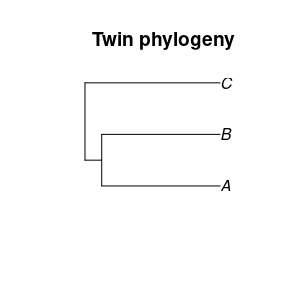### 1.6 Create a twin tree using a built-in function to copy a tree Now using a copy function: # Create twinning_params twinning_params <- pirouette::create_twinning_params( sim_twin_tree_fun = pirouette::create_copy_twtr_from_true_fun() ) # Create a twin tree using my function twin_tree <- create_twin_tree( phylogeny = true_phylogeny, twinning_params = twinning_params ) # Show the twin tree ape::plot.phylo(twin_tree, main = "Twin phylogeny")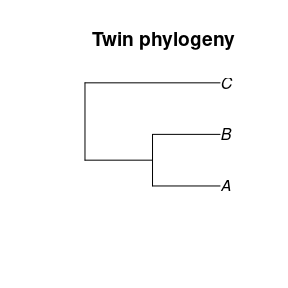Using partial function evaluation you can now plug in any function to create a twin tree! ## 2. create a true alignment We start from a simple true tree: true_phylogeny <- ape::read.tree(text = "((A:1, B:1):1, C:2);") ape::plot.phylo(true_phylogeny, main = "True phylogeny")Also, we’ll specify a root sequence: root_sequence <- pirouette::create_blocked_dna(length = 16) We want to create a true alignment from it. There is a function that does so: alignment_params <- pirouette::create_alignment_params( root_sequence = root_sequence ) true_alignment <- pirouette::sim_true_alignment( true_phylogeny = true_phylogeny, alignment_params = alignment_params ) ape::image.DNAbin(true_alignment, main = "True alignment", legend = FALSE)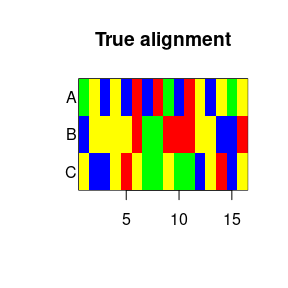How to specify how the true alignment is created? Use the alignment_paramssim_tral_fun:

alignment_params <- pirouette::create_alignment_params(
sim_tral_fun = sim_tral_with_std_nsm,
root_sequence = root_sequence
)
true_alignment <- pirouette::sim_true_alignment(
true_phylogeny = true_phylogeny,
alignment_params = alignment_params
)
ape::image.DNAbin(true_alignment, main = "True alignment", legend = FALSE)Due to this architecture, alignment_paramssim_tral_fun must be a function that takes one argument, which is the true phylogeny. Here is an example that creates a true alignment by simulating a random alignment with the same number of taxa: # My function my_fun <- function( true_phylogeny, root_sequence ) { sequences <- list() for (i in seq_len(ape::Ntip(true_phylogeny))) { sequences[[i]] <- rep( sample(c("a", "c", "g", "t"), size = 1), nchar(root_sequence) ) } ape::as.DNAbin(sequences) } # Putting my function in the alignment_params alignment_params <- pirouette::create_alignment_params( sim_tral_fun = my_fun, root_sequence = root_sequence ) # Simulate the true alignment using my function true_alignment <- pirouette::sim_true_alignment( true_phylogeny = true_phylogeny, alignment_params = alignment_params ) # Show the true alignment ape::image.DNAbin(true_alignment, main = "True alignment", legend = FALSE)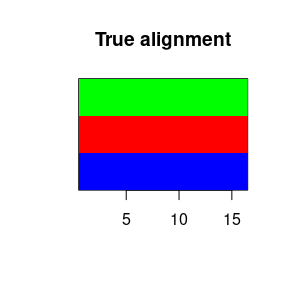So, alignment_paramssim_tral_fun must be a function that takes one argument, which is the true phylogeny. What if we want a function that indeed has one argument for the true phylogeny, but also some additional ones?

An example is sim_tral_with_std_nsm, which uses a root sequence of acgt, a mutation rate of 0.1 and a JC69 site model:

head(sim_tral_with_std_nsm)
##
## 1 function (true_phylogeny, root_sequence, mutation_rate = 1, site_model = beautier::create_jc69_site_model())
## 2 {
## 3     alignment <- pirouette::sim_alignment_with_std_nsm(phylogeny = true_phylogeny,
## 4         root_sequence = root_sequence, mutation_rate = mutation_rate,
## 5         site_model = site_model)
## 6     pirouette::check_alignment(alignment)

To change the default arguments, we’ll need partial function evaluation, which will create a function with one a ellipsis (...) argument that will fill in the values you specified:

sim_tral_fun <- pryr::partial(
sim_tral_with_std_nsm,
mutation_rate = 0.5,
site_model = beautier::create_hky_site_model()
)
head(sim_tral_fun)
##
## 1 function (...)
## 2 sim_tral_with_std_nsm(mutation_rate = 0.5, site_model = beautier::create_hky_site_model(),
## 3     ...)
alignment_params <- pirouette::create_alignment_params(
sim_tral_fun = sim_tral_fun,
root_sequence = root_sequence
)
true_alignment <- pirouette::sim_true_alignment(
true_phylogeny = true_phylogeny,
alignment_params = alignment_params
)
ape::image.DNAbin(true_alignment, main = "True alignment", legend = FALSE)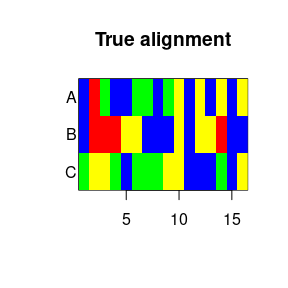Instead of doing the partial function evaluation yourself, pirouette supplies this function as get_sim_tral_with_std_nsm_fun:

alignment_params <- pirouette::create_alignment_params(
sim_tral_fun =
pirouette::get_sim_tral_with_std_nsm_fun(
mutation_rate = 0.5,
site_model = beautier::create_hky_site_model()
),
root_sequence = root_sequence
)
true_alignment <- pirouette::sim_true_alignment(
true_phylogeny = true_phylogeny,
alignment_params = alignment_params
)
ape::image.DNAbin(true_alignment, main = "True alignment", legend = FALSE)Or to use the linked node substitution model, use get_sim_tral_with_lns_nsm_fun:

if (1 == 2) { # nodeSub is not yet on CRAN
alignment_params <- pirouette::create_alignment_params(
sim_tral_fun =
get_sim_tral_with_lns_nsm_fun(
branch_mutation_rate = 0.1,
node_mutation_rate = 0.2
),
root_sequence = root_sequence
)
true_alignment <- pirouette::sim_true_alignment(
true_phylogeny = true_phylogeny,
alignment_params = alignment_params
)
ape::image.DNAbin(true_alignment, main = "True alignment", legend = FALSE)
}

Or to use the unlinked node substitution model, use get_sim_tral_with_uns_nsm_fun:

if (1 == 2) { # nodeSub is not yet on CRAN
alignment_params <- pirouette::create_alignment_params(
sim_tral_fun =
pirouette::get_sim_tral_with_uns_nsm_fun(
branch_mutation_rate = 1.0,
node_mutation_rate = 2.0,
node_time = 0.1
),
root_sequence = root_sequence
)
true_alignment <- pirouette::sim_true_alignment(
true_phylogeny = true_phylogeny,
alignment_params = alignment_params
)
ape::image.DNAbin(true_alignment, main = "True alignment", legend = FALSE)
}

Using partial function evaluation you can now plug in any function to create a true alignment.

## 3. create a twin alignment

To create a twin alignment, we need * a twin tree * a true alignent * a root sequence

Note that some of these three elements may be ignored, but is some cases you will need all three.

As the twin tree, we’ll use this:

twin_phylogeny <- ape::read.tree(text = "((A:2, B:2):1, C:3);")
ape::plot.phylo(twin_phylogeny, main = "Twin phylogeny")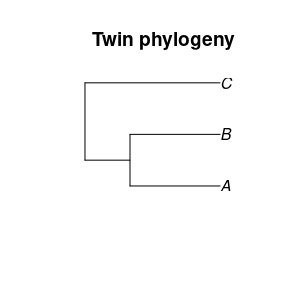The root sequence is short and simple:

root_sequence <- pirouette::create_blocked_dna(length = 20)

As a true alignment, we’ll use something equally simple:

true_alignment <- pirouette::get_test_alignment(
n_taxa = ape::Ntip(true_phylogeny),
sequence_length = nchar(root_sequence)
)
ape::image.DNAbin(true_alignment, main = "True alignment", legend = FALSE)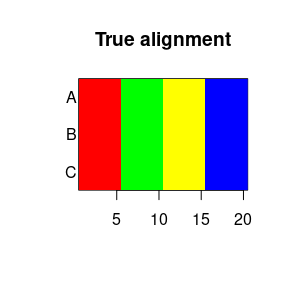I will show multiple ways to create a twin alignment here:

• 3.1. Copy the true alignment
• 3.2. Simulate an alignment based on the twin tree
• 3.3. Simulate an alignment based on the twin tree, true alignment and root sequence

## 3.1. Copy the true alignment

The function we’ll use has three parameters and ignores two:

my_fun <- function(
twin_phylogeny = "irrelevant",
true_alignment,
root_sequence = "irrelevant"
) {
true_alignment
}

Put this function in the twinning_params:

twinning_params <- pirouette::create_twinning_params(
sim_twal_fun = my_fun
)
twin_alignment <- pirouette::sim_twin_alignment(
twin_phylogeny = twin_phylogeny,
true_alignment = true_alignment,
alignment_params = pirouette::create_test_alignment_params(
root_sequence = root_sequence
),
twinning_params = twinning_params
)
ape::image.DNAbin(twin_alignment, main = "Twin alignment")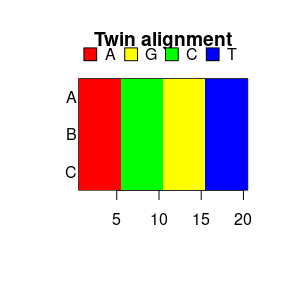## 3.2. Simulate an alignment based on the twin tree

Now we want to plug in:

head(sim_twal_with_std_nsm)
##
## 1 function (twin_phylogeny, root_sequence, true_alignment = "irrelevant",
## 2     mutation_rate = 1, site_model = beautier::create_jc69_site_model())
## 3 {
## 4     alignment <- pirouette::sim_alignment_with_std_nsm(phylogeny = twin_phylogeny,
## 5         root_sequence = root_sequence, mutation_rate = mutation_rate,
## 6         site_model = site_model)

Here comes partial function evaluation to the rescue:

sim_twin_align_fun <- pryr::partial(
sim_twal_with_std_nsm,
mutation_rate = 0.1
)
head(sim_twin_align_fun)
##
## 1 function (...)
## 2 sim_twal_with_std_nsm(mutation_rate = 0.1, ...)
pirouette::check_sim_twal_fun(sim_twin_align_fun)

Plugging it in:

twin_alignment <- pirouette::sim_twin_alignment(
twin_phylogeny = twin_phylogeny,
true_alignment = true_alignment,
alignment_params = pirouette::create_test_alignment_params(),
twinning_params = pirouette::create_twinning_params(
sim_twal_fun = sim_twin_align_fun
)
)
ape::image.DNAbin(twin_alignment, main = "Twin alignment", legend = FALSE)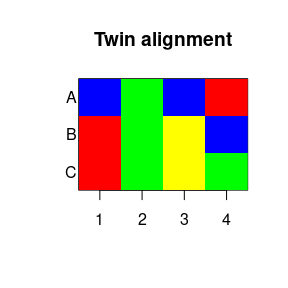Or use get_sim_twal_with_std_nsm_fun to do the partial function evaluation for you:

twin_alignment <- pirouette::sim_twin_alignment(
twin_phylogeny = twin_phylogeny,
true_alignment = true_alignment,
alignment_params = pirouette::create_test_alignment_params(),
twinning_params = pirouette::create_twinning_params(
sim_twal_fun =
pirouette::get_sim_twal_with_std_nsm_fun(
mutation_rate = 0.1
)
)
)
ape::image.DNAbin(twin_alignment, main = "Twin alignment", legend = FALSE)## 3.3. Simulate an alignment based on the twin tree, true alignment and root sequence

Now we want to plug in:

head(sim_twal_with_same_n_mutation)
##
## 1 function (twin_phylogeny, true_alignment, root_sequence, mutation_rate = 1,
## 2     site_model = beautier::create_jc69_site_model(), max_n_tries = 1000,
## 3     verbose = FALSE)
## 4 {
## 5     beautier::check_phylogeny(twin_phylogeny)
## 6     pirouette::check_alignment(true_alignment)

Here comes partial function evaluation to the rescue:

sim_twin_align_fun <- pryr::partial(
sim_twal_with_same_n_mutation,
mutation_rate = 0.5
)
head(sim_twin_align_fun)
##
## 1 function (...)
## 2 sim_twal_with_same_n_mutation(mutation_rate = 0.5, ...)
pirouette::check_sim_twal_fun(sim_twin_align_fun)

Plugging it in:

twin_alignment <- pirouette::sim_twin_alignment(
twin_phylogeny = twin_phylogeny,
true_alignment = true_alignment,
alignment_params = pirouette::create_test_alignment_params(
root_sequence = root_sequence
),
twinning_params = pirouette::create_twinning_params(
sim_twal_fun = sim_twin_align_fun
)
)
## Warning in pirouette::sim_alignment_with_n_mutations(phylogeny =
## twin_phylogeny, : 'sim_alignment_with_n_mutations' tried 1000 times, without
## simulating an alignment with 0 mutations
ape::image.DNAbin(twin_alignment, main = "Twin alignment", legend = FALSE)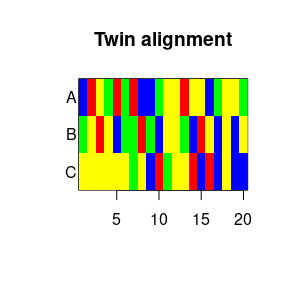Or use get_sim_twal_with_std_nsm_fun to do the partial function evaluation for you:

twin_alignment <- pirouette::sim_twin_alignment(
twin_phylogeny = twin_phylogeny,
true_alignment = true_alignment,
alignment_params = pirouette::create_test_alignment_params(),
twinning_params = pirouette::create_twinning_params(
sim_twal_fun =
pirouette::get_sim_twal_with_std_nsm_fun(
mutation_rate = 0.1
)
)
)
ape::image.DNAbin(twin_alignment, main = "Twin alignment", legend = FALSE)